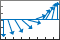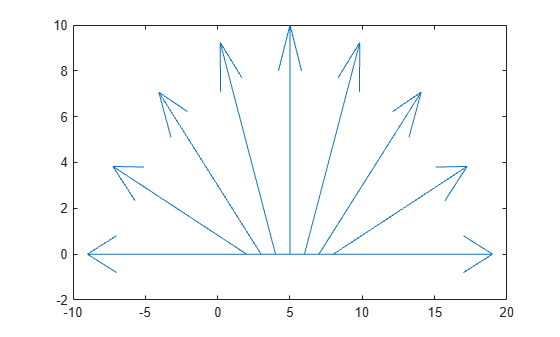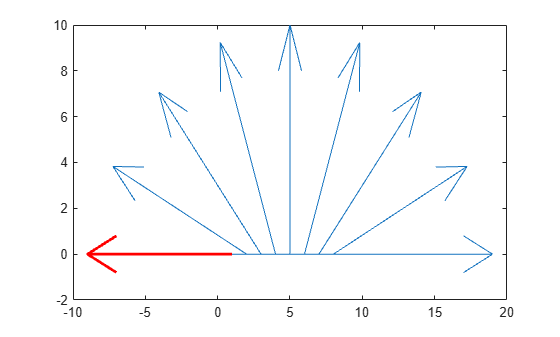# feather

Arrows from x-axis

•## Syntax

``feather(U,V)``
``feather(Z)``
``feather(___,LineSpec)``
``feather(ax,___)``
``f = feather(___)``

## Description

example

````feather(U,V)` plots arrows originating from the x-axis. Specify the direction of arrows using the Cartesian components `U` and `V`, with `U` indicating the x-components and `V` indicating the y-components. The nth arrow has its base at n on the x-axis. The number of arrows matches the number of elements in `U` and `V`.```

example

````feather(Z)` plots arrows using the complex values specified by `Z`, with the real part indicating the x-components and the imaginary part indicating the y-components. This syntax is equivalent to `feather(real(Z),imag(Z))`.```

example

````feather(___,LineSpec)` sets the line style, marker symbol, and color for the arrows.```

example

````feather(ax,___)` plots arrows in the specified axes instead of the current axes.```

example

````f = feather(___)` returns a vector of `Line` objects with `length(U)+1` elements. The first `length(U)` elements represent individual arrows, and the last element represents a horizontal line along the x-axis. Use these `Line` objects to control the appearance of the plot after creating it.```

## Examples

collapse all

Create a feather plot by specifying the components of each arrow as Cartesian values. The nth arrow originates from n on the x-axis.

```t = -pi/2:pi/8:pi/2; u = 10*sin(t); v = 10*cos(t); feather(u,v)```Create a feather plot using polar coordinates by first converting them to Cartesian coordinates.

To do this, create vectors with polar coordinates. Convert them to Cartesian coordinates using the `pol2cart` function. Then, create the plot.

```th = -pi/2:pi/16:0; r = 10*ones(size(th)); [u,v] = pol2cart(th,r); feather(u,v)```Create a vector of complex values. Then, display them using a feather plot. The real part determines the x-component of each arrow, and the imaginary part determines the y-component.

```Z = [2+3i -1-3i -1+i 2i 3-4i -2-2i -2+4i 0.5-i -3i 1+i]; feather(Z)```Create a feather plot with red arrows.

```t = -pi/2:pi/8:pi/2; u = 10*sin(t); v = 10*cos(t); feather(u,v,'r')```Specify the line width and color of a single arrow by assigning the arrow to a variable and then setting its properties. To do this, first create a feather plot and return an array of `Line` objects.

```t = -pi/2:pi/8:pi/2; u = 10*sin(t); v = 10*cos(t); f = feather(u,v);```

Assign the first arrow to a variable. The first arrow corresponds to the first elements of `u` and `v`. Then, change the line width and color.

```f1 = f(1); f1.Color = 'r'; f1.LineWidth = 2;```Starting in R2019b, you can display a tiling of plots using the `tiledlayout` and `nexttile` functions. Call the `tiledlayout` function to create a 1-by-2 tiled chart layout. Call the `nexttile` function to create an axes object and return the object as `ax1`. Create the left plot by passing `ax1` to the `feather` function. Add a title to the plot by passing the axes to the `title` function. Repeat the process to create the right plot.

```tiledlayout(1,2) % Left plot ax1 = nexttile; t = 0:pi/8:pi/2; u1 = 10*sin(t); v1 = 10*cos(t); feather(ax1,u1,v1) title(ax1,'Left Plot') % Right plot ax2 = nexttile; u2 = zeros(5,1); v2 = [1 -2 3 -4 5]; feather(ax2,u2,v2) title(ax2,'Right Plot')```## Input Arguments

collapse all

x-components, specified as a scalar, vector, or matrix. Specify Cartesian values. To convert data from polar to Cartesian, use `pol2cart`.

The size of `U` must match the size of `V`.

y-components, specified as a scalar, vector, or matrix. Specify Cartesian values. To convert data from polar to Cartesian, use `pol2cart`.

The size of `V` must match the size of `U`.

Complex values, specified as a scalar, vector, or matrix. The real part of `Z` indicates the x-components of arrows, and the imaginary part indicates the y-components.

Line style, marker, and color, specified as a character vector or string containing symbols. The symbols can appear in any order. You do not need to specify all three characteristics (line style, marker, and color). For example, if you omit the line style and specify the marker, then the plot shows only the marker and no line.

Example: `'--or'` is a red dashed line with circle markers

Line StyleDescriptionResulting Line
`'-'`Solid line`'--'`Dashed line`':'`Dotted line`'-.'`Dash-dotted lineMarkerDescriptionResulting Marker
`'o'`Circle`'+'`Plus sign`'*'`Asterisk`'.'`Point`'x'`Cross`'_'`Horizontal line`'|'`Vertical line`'s'`Square`'d'`Diamond`'^'`Upward-pointing triangle`'v'`Downward-pointing triangle`'>'`Right-pointing triangle`'<'`Left-pointing triangle`'p'`Pentagram`'h'`HexagramColor NameShort NameRGB TripletAppearance
`'red'``'r'``[1 0 0]``'green'``'g'``[0 1 0]``'blue'``'b'``[0 0 1]``'cyan'` `'c'``[0 1 1]``'magenta'``'m'``[1 0 1]``'yellow'``'y'``[1 1 0]``'black'``'k'``[0 0 0]``'white'``'w'``[1 1 1]`Target axes, specified as an `Axes` object.

## Version History

Introduced before R2006a